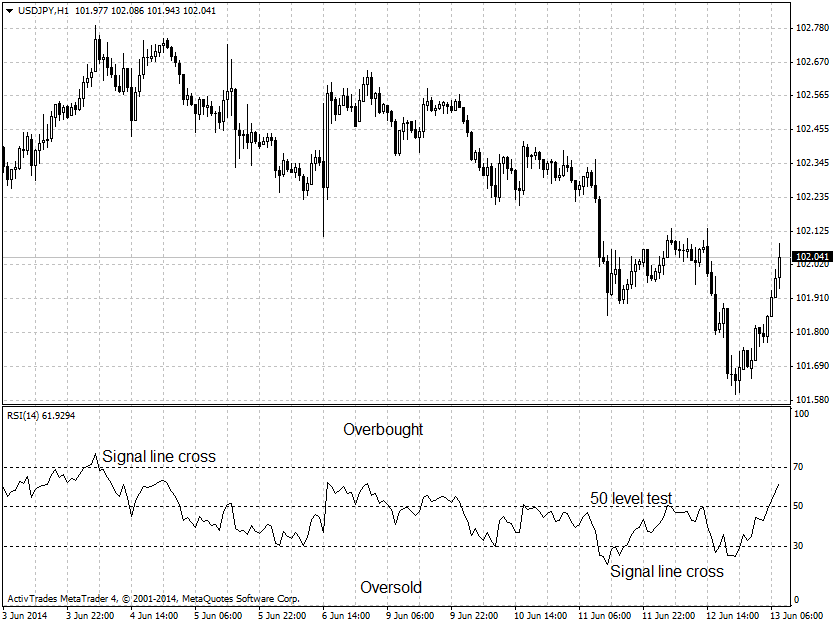# forex software

Create and Test Forex Strategies

forex software

trading:rsi

# Relative Strength Index - RSI

The relative strength index (RSI) is designed to show the current and historical strength or weakness of the market based on the closing prices of a recent trading period. This is a momentum oscillator, measuring the velocity and magnitude of directional price movements. RSI is calculated as the ratio of higher closes to lower closes by the following formula:

`  RSI = 100 - 100/(1 + RS)`
`  RS = Average of last "n" periods up closes / Average of last "n" periods down closes`

RSI is plotted on a scale from 0 to 100 with main signal levels 30 and 70. Some traders and analysts use the 50 level for trading signals during a trend.

RSI is generating the following signals:

• Above 70 - overbought conditions
• Below 30 - oversold conditions
• Crosses of signal lines
• Divergence of the price and indicator charts
• Test of the 50 level during a correction of the trend